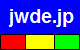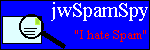joewein.de LLC
fighting spam and scams on the Internet
 Try our spam filter! Free trial for 30 daysHome About Us Spam 419/Nigeria Fraud Contact

### "419" Scam – Advance Fee / Fake Lottery Scam

The so-called "419" scam is a type of fraud dominated by criminals from Nigeria and other countries in Africa. Victims of the scam are promised a large amount of money, such as a lottery prize, inheritance, money sitting in some bank account, etc.

Victims never receive this non-existent fortune but are tricked into sending their money to the criminals, who remain anonymous. They hide their real identity and location by using fake names and fake postal addresses as well as communicating via anonymous free email accounts and mobile phones.

Keep in mind that scammers DO NOT use their real names when defrauding people.
The criminals either abuse names of real people or companies or invent names or addresses.
Any real people or companies mentioned below have NO CONNECTION to the scammers!

Read more about such scams here or in our 419 FAQ. Use the Scam-O-Matic to verify suspect emails.

• This email message is a fake lottery scam. Consider the following facts about real lotteries:
1. They don't notify winners by email.
2. You can't win without first buying a lottery ticket.
3. They don't randomly select email addresses to award prizes to.
4. They don't use free email accounts (Yahoo, Hotmail, etc) to communicate with you.
5. They don't tell you to call a mobile phone number.
6. They don't tell you to keep your winnings secret.
7. They will never ask a winner to pay any fees to receive a prize!
• This email lists mobile phone numbers. Use of such numbers is typical for scams because they allow criminals to conceal their true location. They can receive calls in an Internet cafe from where they send you emails, while pretending to be in some office.
• +448709748431 (UK, redirects to a mobile phone in another country)

Fraud email example:

From: "MRS MEG McMILLIAN'S OFFICE" <megmilly12@hotmail.com>
Date: Thu, 1 May 2008 23:17:02 -0400
Subject:
=?windows-1256?Q?ATTN_;Mr._H._A._van_Huisstede(YOU_HAVE_BEEN_VERIFIED_TO_?=
=?windows-1256?Q?CONTACT_OUR_BANK)=FE=FE=FE=FE=FE=FE=FE=FE=FE=FE=FE=FE=FE?=
=?windows-1256?Q?=FE=FE=FE?=

Ref: UK/7803XI/04Batch: 03/BT25/0306
ATTN ;Mr. H. A. van Huisstede
RE: Referral Code: EQ36Y07I write to inform you of the receipt of your cl=
aims form. However, after a careful consideration and verification of the=
information, we have gone ahead to process your claims and I am very ple=
ased to inform you that approval has been given and our corresponding ban=
k has been advised accordingly.Further to your application of claim of yo=
ur lottery prize awards winning be further advised that your application =
for payment has been approved for immediate remittance of your funds via =
International swift transfer/Bank certified draft as cash payment is mean=
t for resident winners only and our corresponding bank has been advised a=
ccordingly.Find here with the contact information of our corresponding ba=
nk quoting your referral code: Pls note that the bank MUST be contacted a=
s soon as you get this email , through the emails below=3D=3D=3D=3D=3D=3D=
=3D=3D=3D=3D=3D=3D=3D=3D=3D=3D=3D=3D=3D=3D=3D=3D=3D=3D=3D=3D=3D=3D=3D=3D=3D=
=3D=3D=3D=3D=3D=3D=3D=3D=3D=3D=3D=3D=3D=3D=3D=3D=3D=3D=3D=3D=3D=3D=3D=3D=3D=
=3D=3D=3D=3D=3D=3D=3D=3D=3D=3DBUTTERFIELD PRIVATE BANK TEL: +44 702 402 3=
376,+44 702 402 0893 FAX: +44 870 974 8431 EMAIL: customercare_bfpbank@ya=
hoo.co.uk, CONTACT: MR PETER HOWARD=3D=3D=3D=3D=3D=3D=3D=3D=3D=3D=3D=3D=3D=
=3D=3D=3D=3D=3D=3D=3D=3D=3D=3D=3D=3D=3D=3D=3D=3D=3D=3D=3D=3D=3D=3D=3D=3D=3D=
=3D=3D=3D=3D=3D=3D=3D=3D=3D=3D=3D=3D=3D=3D=3D=3D=3D=3D=3D=3D=3D=3D=3D=3D=3D=
=3D=3D=3D So please you are advised to contact the bank with effect from=
today with the above email/phone/fax informing them that you have been r=
eferred by Mrs. Meg McMillian, You must not forget to quote the above ref=
erral code in your email/fax/call to them becauseyour file will be traced=
by the code. You should also send your details just to verify what they =
if you need further assistance.NB; Your amount won is 500 000GBPYour Bat=
ch and Ref Numbers are Batch: 03/BT25/0306 & Ref: UK/7803XI/04 The follow=
ing requirements should be sent to their offices for confirmation and fin=
al process for payment.=3D=3D=3D=3D=3D=3D=3D=3D=3D=3D=3D=3D=3D=3D=3D=3D=3D=
=3D=3D=3D=3D=3D=3D=3D=3D=3D=3D=3D=3D=3D=3D=3D=3D=3D=3D=3D=3D=3D=3D=3D=3D=3D=
=3D=3D=3D=3D=3D=3D=3D=3D=3D=3D=3D=3D=3D=3D=3D=3D=3D=3D=3D=3D=3D1, Referen=
ce Number2, Batch Number3, Full Names*4, Bank details .{IMPORTANT}5, A me=
dium of identification, ie either a copy ofdrivers license or internation=
al passport or any otherlegal means of identification.{IMPORTANT}6, Amoun=
t Won.7, Contact Phone Numbers=3D=3D=3D=3D=3D=3D=3D=3D=3D=3D=3D=3D=3D=3D=3D=
=3D=3D=3D=3D=3D=3D=3D=3D=3D=3D=3D=3D=3D=3D=3D=3D=3D=3D=3D=3D=3D=3D=3D=3D=3D=
=3D=3D=3D=3D=3D=3D=3D=3D=3D=3D=3D=3D=3D=3D=3D=3D=3D=3D=3D=3D=3D=3D=3D=3DU=
pon submission of the required information as stated to their offices, yo=
u will be cleared for payment from their offices.Note: No deduction whats=
d to avoid fraudulent activities. You are responsible for the transfer c=
hargesfrom their courier offices. If you do not pay the transfer charges =
from their offices, your funds will not be transfered to your bank accoun=
t. Please be informed. You are advisedto comply with their delivery rules=
and regulations in order to receive your winnings as prompt as possible.=
Once again, we extend a warm congratulation to you for your lucky prize a=
wards winning which your fate has brought to you and hope you shall be wi=
er inquiry and assistance (IF ANY). We are at your complete disposal for =
efficient and reliable services.Yours Faithfully,Mrs. Meg McMillian=20

You must be 16 or over to play or claim a=20
_________________________________________________________________
Make i'm yours.=A0 Create a custom banner to support your cause.
http://im.live.com/Messenger/IM/Contribute/Default.aspx?source=3DTXT_TAGH=
M_MSN_Make_IM_Yours

Anti-fraud resources: